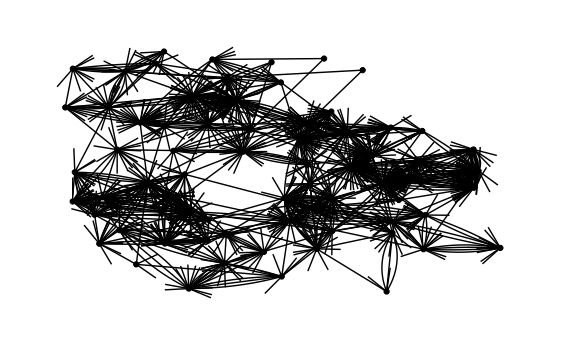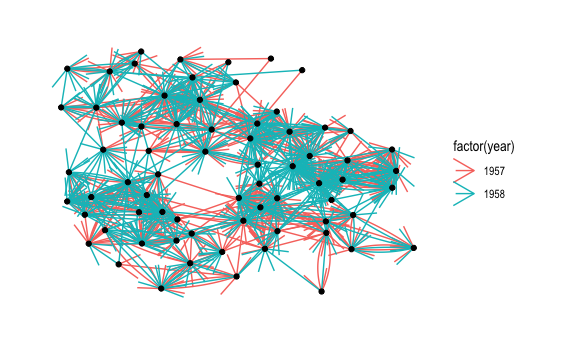``````library(tidygraph)
library(ggraph)
# annotate highschool graph example
# as a tidygraph
data(highschool)
graph <- as_tbl_graph(highschool)``````

Plot the graph as is

``````graph %>%
ggraph(layout="kk") +
geom_edge_fan(arrow=arrow()) +
geom_node_point() +
theme_graph(foreground=NA)``````Print the graph object

``graph``
``````# A tbl_graph: 70 nodes and 506 edges
#
# A directed multigraph with 1 component
#
[90m# Node Data: 70 x 1 (active)[39m
name
[3m[90m<chr>[39m[23m
[90m1[39m 1
[90m2[39m 2
[90m3[39m 3
[90m4[39m 4
[90m5[39m 5
[90m6[39m 6
[90m# ... with 64 more rows[39m
#
[90m# Edge Data: 506 x 3[39m
from    to  year
[3m[90m<int>[39m[23m [3m[90m<int>[39m[23m [3m[90m<dbl>[39m[23m
[90m1[39m     1    13  [4m1[24m957
[90m2[39m     1    14  [4m1[24m957
[90m3[39m     1    20  [4m1[24m957
[90m# ... with 503 more rows[39m``````

Use edge color to indicate year

``````graph %>%
ggraph(layout="kk") +
geom_edge_fan(aes(color=factor(year)), arrow=arrow()) +
geom_node_point() +
theme_graph(foreground=NA)``````Let’s only look at the 1958 subgraph

``````graph %>%
activate(edges) %>%
filter(year == 1958) %>%
ggraph(layout="kk") +
geom_edge_fan(aes(color=factor(year)), arrow=arrow()) +
geom_node_point() +
theme_graph(foreground=NA)``````